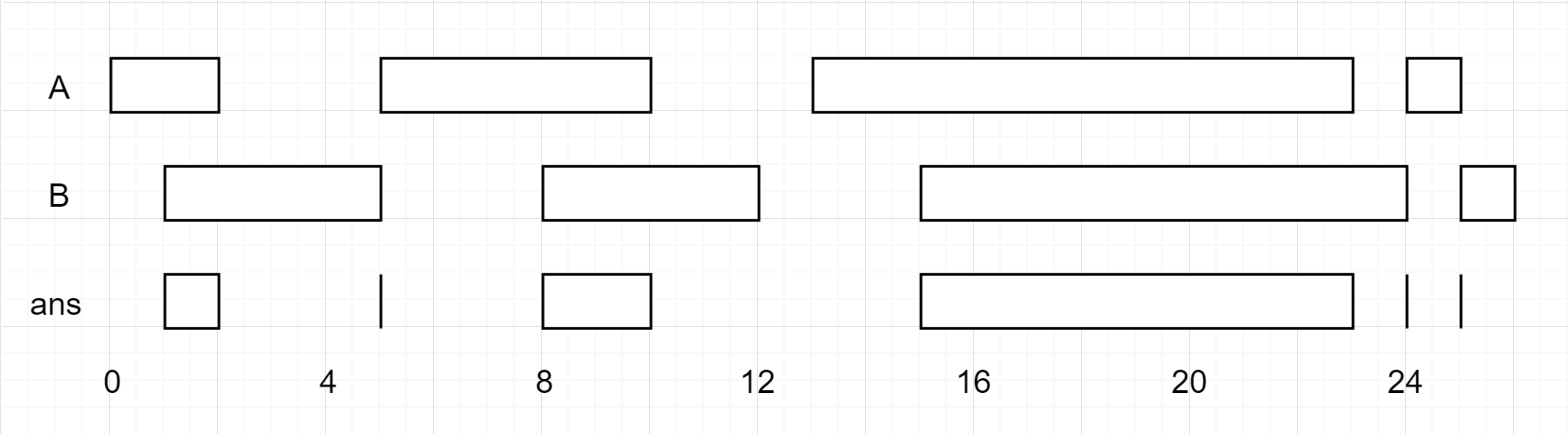## 1.1. 题目

### 1.1.1. 区间列表的交集``````输入：firstList = [[0,2],[5,10],[13,23],[24,25]], secondList = [[1,5],[8,12],[15,24],[25,26]]

``````

``````输入：firstList = [[1,3],[5,9]], secondList = []

``````

``````输入：firstList = [], secondList = [[4,8],[10,12]]

``````

``````输入：firstList = [[1,7]], secondList = [[3,10]]

``````

### 1.1.2. 题解:

• 两个有序的二维数组,然后求交集
• 交集是集合,也可以是某个整数点,如果是同一个点,可以用`[]int{n,n}`表示两个区间无交集,比如`{1,2},{2,3}`是不允许的

### 1.1.3. 思路:

• 第一反应是双指针,慢慢的往后遍历两个集合
• 另外,两个数组的交集,比如 `[]int{1,10}``[]int{2,11}` 是 集合的最小值的最大值 与 集合的最大值的最小值
• 如果 最小值的最大值 大于 最大值的最小值,那说明集合没有相交,
• 比如 上面例子 `{1,10} 和 {2,11}`
• 最小值的最大值是 `2`
• 最大值的最小值是 `10`
• 2<10 所以是有效的交集
• 那么,指针应该怎么挪呢, 比如 `{1,3}``{4,6}`,他俩无交集, 很明显, `{1,3}`这个指针应该往后挪,因为 3 < 6

### 1.1.4. 代码:

``````
func intervalIntersection(firstList [][]int, secondList [][]int) [][]int {
// 正常有一个为空,就不需要求了
if len(firstList) == 0 || len(secondList) == 0 {
return nil
}

// 定义两个指针移动
var i, j int
// 定义一个交集
var re [][]int
// 当指针都移动到了各自的末尾,就结束循环
for len(firstList) > i && len(secondList) > j {
// 合并两个数组的交集
res := combine(firstList[i], secondList[j])
// 如果有交集,放到交集列表里
if res != nil {
re = append(re, res)
}

// 判断谁该往后挪, 可以看上面题解
// 谁比较小,就谁挪
if firstList[i] < secondList[j] {
i++
} else {
j++
}

}
return re
}

func combine(a, b []int) (r []int) {

// 定义最小值的最大值,和最大值的最小值
var minMax, maxMin int
if a > b {
minMax = a
} else {
minMax = b
}

if a > b {
maxMin = b
} else {
maxMin = a
}
// 毫无相交
if minMax > maxMin {
return nil
}

return []int{minMax, maxMin}
}
``````